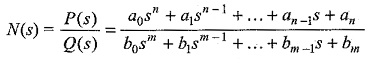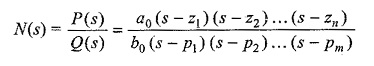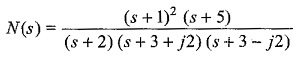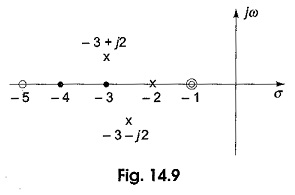## Poles and Zeros of Transfer Function:

Poles and Zeros of Transfer Function defines that, in general, the network function N(s) may be written aswhere a0,a1,…a2 and b0,b1,…bm are the coefficients of the polynomials P(s) and Q(s); they are real and positive for a passive network. If the numerator and denominator of polynomial N(s) are factorized, the network function can be written aswhere z1,z2,…zn are the n roots for P(s)= 0

and p1,p2,…pm are the m roots for Q(s) = 0

and a0/b0 = H is a constant called the scale factor.

z1,z2,…zn in the transfer function are called zeros, and are denoted by 0. Similarly, p1,p2,…pm are called poles, and are denoted by x. The network function N(s) becomes zero whens is equal to anyone of the zeros. N(s) becomes infinite when s is equal to any one of the poles. The network function is completely defined by its Poles and Zeros of Transfer Function. If the poles or zeros are not repeated, then the function is said to be having simple poles or simple zeros. If the poles or zeros are repeated, then the function is said to be having multiple poles multiple zeros. When n > m, then (n — m) zeros are at s = ∞, and for m > n, (m — n) poles are at s = ∞.

Consider, the network functionthat has double zeros at s = – 1 and a zero at s = – 5; and three finite poles at s = – 2, s = – 3 + j2, and s = – 3 – j2 as shown in Fig. 14.9.The network function is said to be stable when the real parts of the poles and zeros are negative. Otherwise, the poles and zeros must lie within the left half of the s-plane.

Scroll to Top